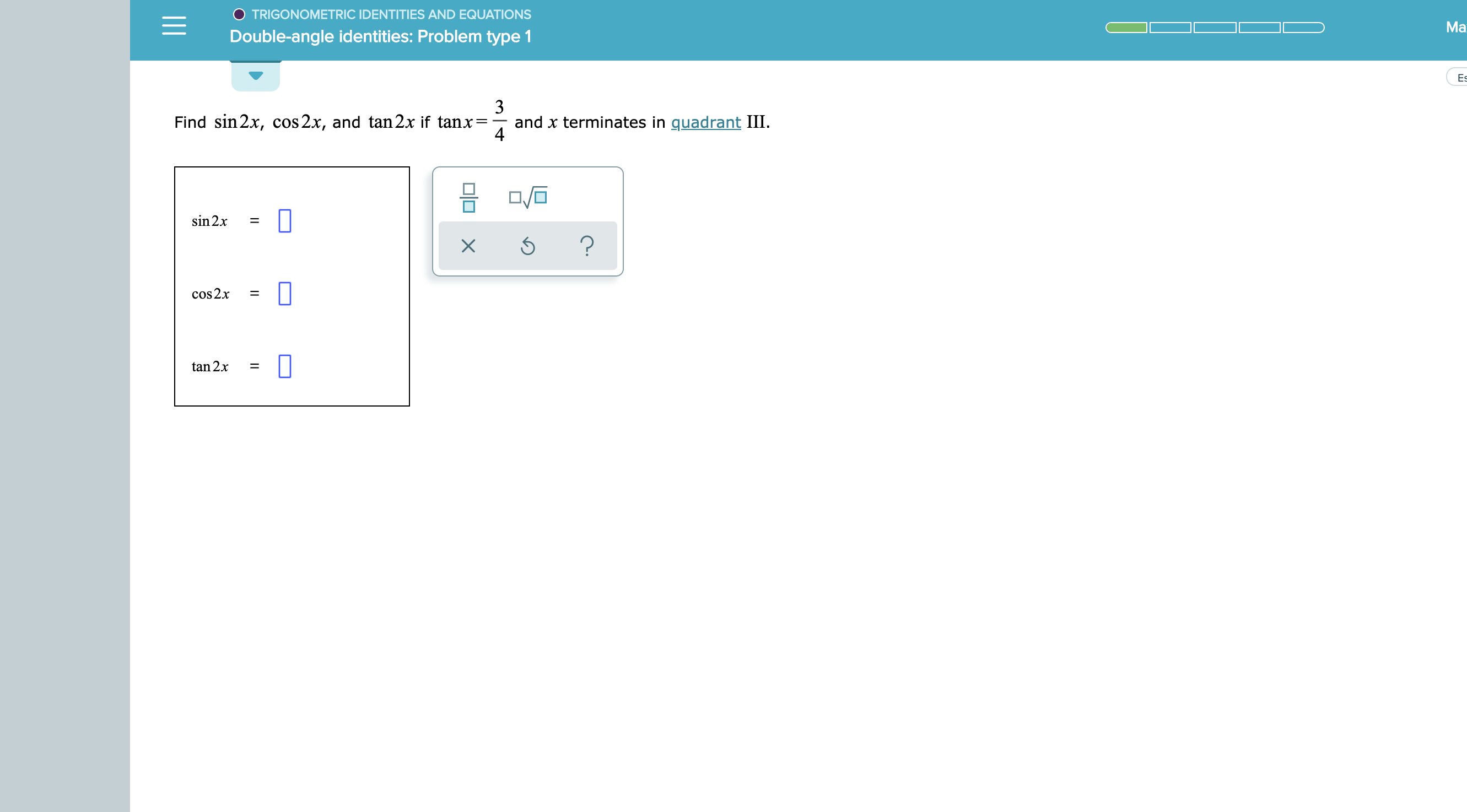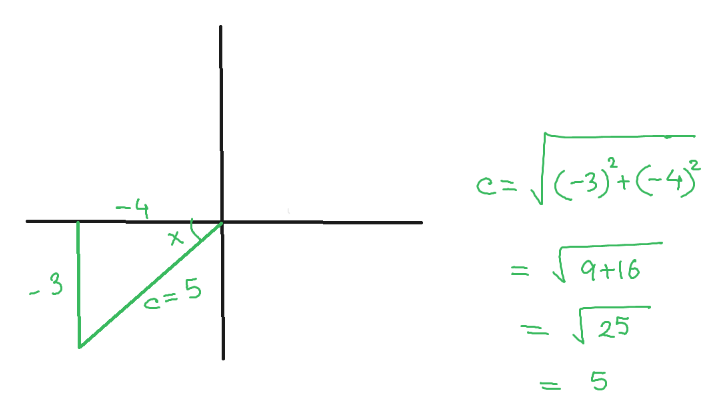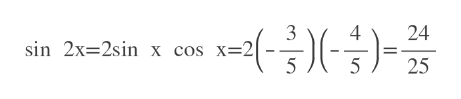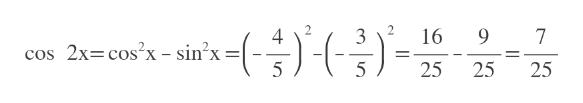O TRIGONOMETRIC IDENTITIES AND EQUATIONSMaDouble-angle identities: Problem type 1Es3Find sin 2x, cos 2x, and tan2x if tanx=and x terminates in quadrant III4sin 2x?cos2x=tan 2x=X

Question

see attachmenthelp_outlineImage TranscriptioncloseO TRIGONOMETRIC IDENTITIES AND EQUATIONS Ma Double-angle identities: Problem type 1 Es 3 Find sin 2x, cos 2x, and tan2x if tanx= and x terminates in quadrant III 4 sin 2x ? cos2x = tan 2x = X fullscreen
Step 1

Since x is in 3rd quadrant, assume opposite of x= -3 and adjacent =-4

So hypotenuse= 5help_outlineImage Transcriptionclose(-3)+ C-4 -4 - 3 9+16 5 25 5 X fullscreen
Step 2

sin x= -3/5 and cos x= -4/5

We use them in double angle formula.

Answer: sin 2x= 24/25help_outlineImage Transcriptionclose3 sin 2x 2sin x cos x=2| 5 24 25 fullscreen
Step 3help_outlineImage Transcriptionclose3 16 9 7 4 cos 2x-cos?x - sin2x 1 25 25 5 25 fullscreen

Want to see the full answer?

See Solution

Want to see this answer and more?

Our solutions are written by experts, many with advanced degrees, and available 24/7

See Solution
Tagged in

Other